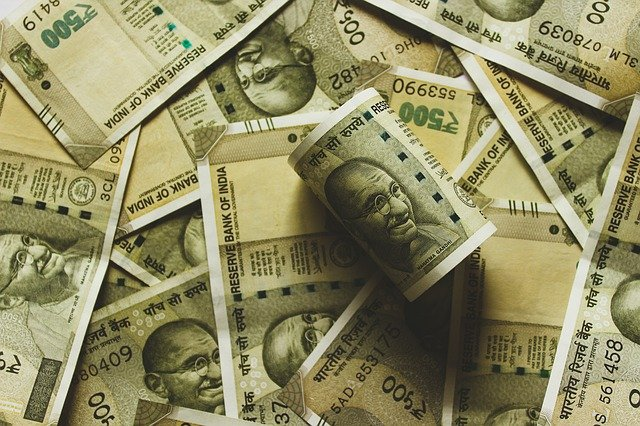# PPF Calculator: Deposit money in PPF with this special trick! Will become the owner of 1.5 crores, understand the calculationPPF Calculator: Public Provident Fund (PPF) is one of the popular options for investment, in which you get better returns. It also saves tax. But, despite being so popular, many times people are not able to take full advantage of it. In such a situation, if you get to know how the interest on PPF is calculated and how you can get maximum interest, then your amount can increase manifold.

Interest rates on PPF have come down only last year

On 30 March 2020, the government had drastically cut the interest rates of small savings schemes. The interest rates on PPF are also at 7.1%. Let us tell you that the interest on small savings schemes and PPF is reviewed every quarter. These interest rates have a big impact on the rate of inflation.

This is how a fund of 1.5 crores will be made

You can invest a maximum of Rs 1.50 lakh in a PPF account in a year. Suppose, you invest Rs 12,500 every month in a PPF account. After maturity in 15 years, you can extend your PPF account in blocks of 5-5 years. In such a situation, after 30 years, the entire fund of your PPF account will be more than 1.5 crores (1,54,50,911). In this, your investment will be 45 lakhs and interest income will be around Rs 1.09 crore.

You can start investing in 25 years

Let us tell you that the sooner you start investing in this government scheme, the more it benefits. Suppose you are 25 years old and you invest Rs 1.5 lakh annually in PPF, So at the age of 55, ie about 5 years before retirement, you can become a millionaire.

How is interest calculated on PPF?

Interest is calculated every month on PPF, but it is credited to the account at the end of the financial year. That is, whatever interest you earn every month is put in your PPF account on 31st March. However, there is no fixed date for when to deposit money in the PPF account. You can deposit money in PPF monthly, quarterly, half yearly and annually.

How to get higher interest on PPF

Let us now explain how interest is calculated. Interest on PPF is calculated on the amount in the account from 1st to 5th of every month. That is, if you put money in the PPF account by the 5th of any month, then interest will be available on that money in the same month, but if you deposited the money after the 5th ie on the 6th, then the interest on the deposited amount will be available in the next month. will get.

Simple Example of PPF Calculation

Let us understand this PPF calculation with an easy example. By which you will come to know how you can get more interest by investing money at the right time.

Example No.-1

Suppose you deposited Rs 50,000 in your account on 5th April, you already have Rs 10 lakhs in your account as of 31st March. From April 5 to April 30, the total amount in your PPF account was Rs 10,50,000, which is the minimum balance. So the monthly interest on it at the rate of 7.1% is – (7.1%/12 X 1050000) = Rs 6212

Example number-2

Now suppose you did not deposit the amount of Rs 50000 till 5th April and then deposited it on 6th April. From April 5 to April 30, the minimum balance in your account will be Rs 10 lakh. What is the monthly interest on this at the rate of
7.1% (7.1%/12 X 10,00,000) = Rs 5917

If you deposit with this trick, you will get more interest

Imagine, the investment amount is only 50,000, but the method of deposit made a difference in the interest. In such a situation, if you want maximum interest on your money in PPF, then keep this trick in mind and deposit the money by the 5th of the month so that you definitely get the interest for that month. Experts also recommend that the investment of 1.5 lakh on PPF is tax-exempt, so if you want to take this tax exemption, then deposit the entire amount of 1.5 lakh between April 1 and April 5 as soon as the new financial year starts. Give. If you are not able to do this, then deposit the money by the 5th of every month.